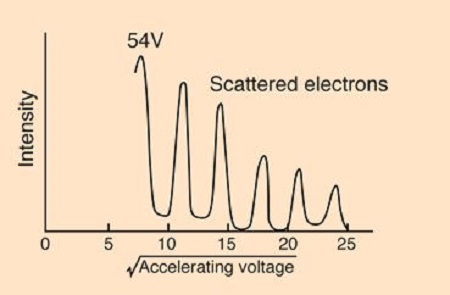# Davisson and Germer experiment : If electrons were considered as particles, draw a graph of the...

## Question:

Davisson and Germer experiment :

If electrons were considered as particles, draw a graph of the number of scattered electrons vs incident angle if the electron gun was positioned at 45 degrees. (how would it be different from the graph of the number of scattered electrons vs incident angle from the Davisson and Germer experiment?)

## Davisson-Germer Experiment:

This experiment showed that particle, specifically electrons, could display a wave-like nature to their movements. This helped to prove deBroglie's idea that all matter could behave like a wave.

Become a Study.com member to unlock this answer!

So to better explain this, let's start with the actual results of the experiment.Here the peaks you see are caused by the electrons leaving the...Wave-Particle Duality & the Davisson-Germer Experiment

from

Chapter 23 / Lesson 2
5.5K

Wave-particle duality means that light and sub-atomic particles can also behave as waves, hence the duality. Study the definition of wave-particle duality, the Davisson-Germer Experiment, the de Broglie wavelength, and an example calculation.